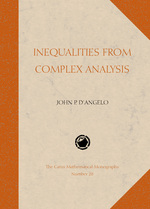Have library access? Log in through your library# Inequalities from Complex Analysis

John P. D’Angelo
Volume: 28
Copyright Date: 2002
Edition: 1
Pages: 281
https://www.jstor.org/stable/10.4169/j.ctt5hh8s7

## Table of Contents

1. Front Matter
(pp. i-vi)
2. Table of Contents
(pp. vii-x)
3. Preface
(pp. xi-xvi)
John P. D’Angelo
4. CHAPTER I Complex Numbers
(pp. 1-32)

Mathematical analysis requires a thorough study of inequalities. This book will discuss inequalities and related positivity conditions arising from complex analysis, in both one and several variables. The statements, proofs, and applications of these inequalities involve diverse parts of mathematics. We aim to provide a systematic development of this material, beginning with the definition of the complex number field.

In Section I.2 we shall note that the mathematical statement$z \le w$does not make sense whenzandware complex but notrealnumbers; ultimately the inequalities we use in complex analysis must be inequalities about real numbers. Furthermore, many...

5. CHAPTER II Complex Euclidean Spaces and Hilbert Spaces
(pp. 33-62)

Next we extend the ideas we found useful in C to both finite-dimensional and infinite-dimensional vector spaces over C. See Appendix A.1 for the list of axioms for a vector space. Most of the geometric reasoning from Chapter I applies in the setting of Hilbert spaces. One of the main new issues will be the existence of orthogonal projections onto closed subspaces. This notion is related to a version of the Pythagorean theorem. We introduce bounded linear transformations (operators) between Hilbert spaces in this chapter, but postpone results about adjoints and Hermitian forms to Chapter IV.

The Riesz representation lemma...

6. CHAPTER III Complex Analysis in Several Variables
(pp. 63-88)

The material in this chapter intentionally interrupts our theoretical development about Hilbert spaces. There are at least two reasons for this interruption. One reason is that this material is a prerequisite for understanding the Hilbert space${A^2}({B_n})$of square-integrable holomorphic functions on the unit ball; this particular Hilbert space arises in a crucial way in the proof of Theorem VII.1.1, the main result in the book. A second reason is the feeling that abstract material doesn’t firmly plant itself in one’s mind unless it is augmented by concrete material. Conversely the presentation of concrete material benefits from appropriate abstract interludes....

7. CHAPTER IV Linear Transformations and Positivity Conditions
(pp. 89-142)

This book aims to provide a systematic discussion of inequalities that bear on complex analysis. Many of these come from linear algebra and positivity conditions for Hermitian linear transformations. We will prove the basic facts about linear transformations on complex Euclidean spaces, and then generalize them to results about bounded transformations on Hilbert spaces. Linear transformations on Hilbert spaces are also known asoperators, and their study is often calledoperator theory.

We prove the spectral theorem for Hermitian operators in finite dimensions in this chapter. We defer to Chapter V its generalization to compact Hermitian operators on a Hilbert...

8. CHAPTER V Compact and Integral Operators
(pp. 143-174)

This chapter discusses compact operators in detail. Section 1 concerns convergence properties for sequences of bounded operators. We introduce compact operators in Section 2. The main result of the chapter is the spectral theorem for compact Hermitian operators. We provide additional discussion about integral operators, and we close the chapter with a glimpse at singular integral operators. This chapter prepares us for the applications in Chapter VII, where we use facts about compact operators to study positivity conditions for polynomials.

Completeness of the real number system is crucial for doing analysis; without itlimitswould be a useless concept. Similarly,...

9. CHAPTER VI Positivity Conditions for Real-valued Functions
(pp. 175-216)

So far, most of our real-valued functions on${C^n}$have been Hermitian forms such as$\langle Lz,z\rangle$. These are linear inzand in$\overline z$. WhenLis nonnegative definite,$\langle Lz,z\rangle = \parallel \sqrt L (z){\parallel ^2}$; the squared norm makes it evident why the values are nonnegative. In this chapter we consider more general real-valued functions of several complex variables, and we apply some of the ideas from Hermitian linear algebra in this nonlinear setting. Things become more interesting, even for real-valued polynomials.

Section 1 helps motivate the rest of the Chapter by considering positivity conditions for polynomials in one and severalrealvariables. Section 2...

10. CHAPTER VII Stabilization and Applications
(pp. 217-242)

The results in this chapter generally have the same flavor. We are given a polynomial whose values are positive on some set. We show that the polynomial agrees with a quotient of squared norms on that set, thus explaining the positivity. These results are striking applications of Theorem VII.1.1; this stabilization result shows that certain bihomogeneous polynomials are quotients of squared norms. See Remark VII.1.4 for an explanation of the termstabilization.

We state and discuss Theorem VII.1.1 in Section 1, but postpone its proof to Section 6. The proof uses the Hilbert space methods developed in this book; it...

11. CHAPTER VIII Afterword
(pp. 243-246)

This afterword aims to provide some glimpses into research about positivity conditions in complex analysis. The papers mentioned here form an incomplete list, but perhaps will give the interested reader an entry to the research literature.

This proof of Theorem VII.1.1 gives a nice application of the theory of compact operators to a concrete problem about polynomials. The proof here follows the approach from [CD1], where Theorem VII.3.1 also appeared. Theorems VII.4.2 and VII.5.1 come from [D2]. The main assertion in Theorem VII.1.1, that S1) implies S3), goes back to [Q]; the proof there uses the Hilbert spaceA²(Cn,dG)...

12. APPENDIX A
(pp. 247-256)
13. Bibliography
(pp. 257-260)
14. Index
(pp. 261-264)